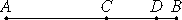# Proposition 26

If an odd number is subtracted from an odd number, then the remainder is even.

Let the odd number BC be subtracted from the odd number AB.

I say that the remainder CA is even.Since AB is odd, subtract the unit BD, therefore the remainder AD is even. For the same reason CD is also even, so that the remainder CA is also even.

Therefore, if an odd number is subtracted from an odd number, then the remainder is even.

Q.E.D.

## Guide

This proposition is used in IX.29.